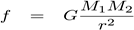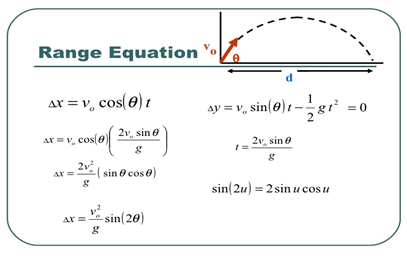# INTRODUCTION

Projectile motion occurs when an object is thrown with a certain velocity in the air in any direction and the only acting force is gravity. Motion on earth has specific principles and limits for the movement of an object in the air, whether it’s inches or thousands of feet off the ground. There are a lot of forces acting on an object when changing direction or just moving. This is how responsive the laws of motion are.

1. No matter what, the net force from all systems will always equal to zero (Newton’s third law).
2. Energy is neither created nor destroyed -the same goes for matter.
3. Rocket propulsion requires the acceleration to be greater than theweight in newtons of the rocket; in other words, it has to be faster than gravity -even in thin air like the exosphere.

There are 5 layers of the atmosphere in the earth’s hemisphere: the troposphere, stratosphere, mesosphere, thermosphere, and exosphere. Every single layer has a different level of atmospheric pressure (similar to the pressure experienced when diving in a deep pool that can crush the body; the same goes for atmospheric pressure but in a gaseous form). This will be covered in another article on Bernoulli’s principle which helps better understand projectile motion for 3-dimensional motion like missiles and rockets.

# Projectile motion in real life

There are many examples of projectile motion, such as missiles, gliders, footballs, and even jumping off a cliff.

In the real world, matrices of these formulas and functions can go between 3 to 4 dimensions (meaning 4 variables of motion),  especially in mid-air where numerous forces are acting on the object. And on the energy side, kinetic energy moves with the object which produces momentum to continue with either accelerating or decelerating relative to the distance ahead.

# The Maths behind it

Vf stands for the final velocity before an object hits the ground, and Vi is the velocity before gravity acts on an object in the air or the thrust in x-direction which moves horizontally.

Xf stands for the height at which an object is, or the range, depending on the projectiles starting and ending motion. X0 can represent X in the y coordinate when graphing this concept.

Just to let you in on a little physics’ secret, you don’t really need different derived formulas to calculate the needed velocity to go to the moon. Projectile motion is how it moves using 3 dimensions. All you do is calculate the strength of gravity outside the earth’s atmosphere which is,then put that number, which will be less than 9.8 m/s2, in the formula which is then to be used as a simple algebraic expression. Next, you can figure out the velocity.The range equation focuses more on starting on y=0, x=0, or basically when the object is the ground; and then, the object gets thrust up till it reaches its maximum height. As gravity takes course, it hits the ground at y=0 but with x the displacement or range in which the object travels doesn’t stay at zero; for example, x=5km.

This is where the formulas are derived from.

# EPILOGUE

Projectile motion equations and formulas, even the concept as a whole, help clarify the motion of an object and becomes a tool similar to how mathematics is a tool for physics; these laws and equations are tools for relative motion and in aerial 3-dimensional motion. Without these discoveries, missiles, planes, bombs, and even formula one racing wouldn’t exist. The best part is the simplicity of Newtonian mechanics which means we don’t need lagrangians and differential calculus when dealing with semi-difficult problems of objects or machines in the air traveling along a path. All we need are these simple systems and sometimes calculus. Projectile motion is the cheat code for modern-day engineers.

References:

1- Ackroyd, James E. Pearson Physics. Pearson, 2018.

2- “Projectile Motion – Definition &amp; Formula: Projectile – Types &amp; Examples.” BYJUS, BYJU’S, 25 Mar. 2021, byjus.com/physics/projectile-motion/.

Writer: Ali F Albati

Editors: Lama Alahdal/Rana Alqahtani